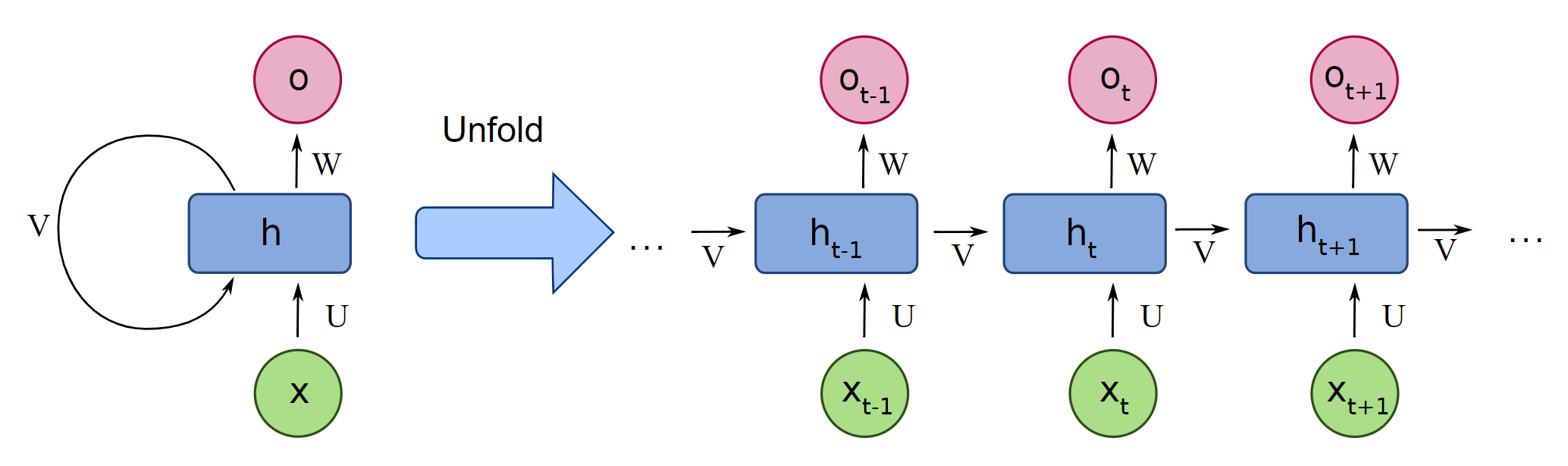# Are recurrent neural networks just vanilla neural networks with sequential data?

Suppose I have a sequence of observations $$x_1, x_2,...,x_n$$. Is fitting a recurrent neural network to this data set the same as fitting a vanilla neural network with inputs $$x_1, x_2, \dots,x_{n-1}$$ and outputs $$x_2, x_3, \dots , x_n$$? If not, what's the difference?

• By "vanilla neural network", are you asking about MLPs? In an MLP, how do you plan to handle varying sequence lengths? – Arya McCarthy Apr 17 at 6:37
• Also—did you intend for both the input and the output to be $x$? You're doing autoregressive sequence modeling? – Arya McCarthy Apr 17 at 6:45

Short answer: no, they're not just vanilla neural networks. There are certainly commonalities, but RNNs can handle tasks that MLPs can't.

I'll begin by quoting some of an answer I gave to this question that proposed using a 'vanilla neural network' for sequence data.

A multi-layer perceptron can only process fixed-size inputs. In your example up above, you could indeed pass [3, 1, 20] (‘cat’) to an MLP if it has 3 input nodes. But you can’t give the MLP [3, 1, 20, 19] (‘cats’)! You’d need to add another input node (and associated weights), then learn the updated weights. Even if you wanted to do this, the number of parameters you need grows with the length of the sequence. At some point, you have to decide ‘enough is enough’ and put a bound on the length of your sequence.

By contrast, an RNN can handle an input sequence (or, depending on your purpose, output sequence) with unbounded length, reusing only a finite number of weights. You don’t need more weights as the sequence gets longer.

So they're different. At the heart, you share this linear-transform-then-squashing pattern, but you apply it differently. In the 'vanilla neural network', you'd consume the entire input sequence at once, transforming it with your weight matrix. In the RNN, you build up a digest of the sequence (the hidden state) from left to right. At each step, the inputs to your matrix are the hidden state (based on the previous input symbols) and the current input symbol.

Because you don't have these long-distance dependencies, the number of parameters is far fewer than in the MLP that takes in $$n - 1$$ symbols and produces $$n - 1$$ symbols. The number of parameters is actually independent of the sequence length.

Finally, let's assume that our input length is fixed, so that your example makes sense. In this circumstance, you don't really need an RNN. Still, it's not the same as an MLP. The MLP lets you use information from the entire input at each point. The RNN, by contrast, only has knowledge of previous symbols in its hidden state, not future ones. (This motivates bidirectional RNNs for encoding sequences.)No, they are not. Recurrent neural network takes as input current timepoint(s) and a hidden state, to generate prediction and a hidden state to be used with next timepoint(s), hence the recursive nature. In vanilla, fully connected networks you treat the inputs independently, so nothing is passed along.